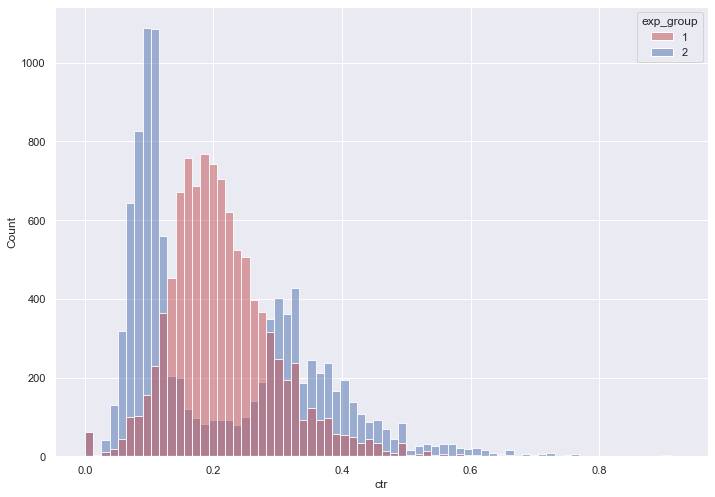I continue my publications on analytics:

There are a huge number of metrics and relationship metrics that can be used for analysis. There are a large number of publications. For example, you can look at Nikita Marshalkin's materials.

In 2018, Yandex researchers developed a method for analyzing tests over metrics $\dfrac{x}{y}$ ratios.

The idea of the method is that instead of pushing "browser-based" CTR into the test, you can construct another metric and analyze it, but at the same time it is guaranteed (unlike smoothed CTR) that if the test on this other metric sees changes, then there are changes in the original metric (that is, in likes per user and in user CTR).

1. We calculate the total CTR in the control group $𝐶𝑇𝑅_{𝑐𝑜𝑛𝑡𝑟𝑜𝑙}=\dfrac{𝑠𝑢𝑚(𝑙𝑖𝑘𝑒𝑠)}{𝑠𝑢𝑚(𝑣𝑖𝑒𝑤𝑠)}$
2. Let's calculate the metric for users in both groups $𝑙𝑖𝑛𝑒𝑎𝑟𝑖𝑧𝑒𝑑𝑙𝑖𝑘𝑒𝑠=𝑙𝑖𝑘𝑒𝑠−𝐶𝑇𝑅_{𝑐𝑜𝑛𝑡𝑟𝑜𝑙} \times 𝑣𝑖𝑒𝑤𝑠$
3. After that, let's compare the differences in the groups by the metric linearizadlikes with the t-test

This simple method guarantees that with a large sample size, it is possible to increase the sensitivity of the metric.

import pandas as pd
import pandahouse as ph
import numpy as np
import matplotlib.pyplot as plt
import seaborn as sns
from scipy import stats

connection = {
'host': 'https://clickhouse.lab.karpov.courses',
'user': '**********',
'database': 'simulator_20220620'
}


The first test will be conducted between groups 0 and 3 according to the metric of linearized likes.

q = """
SELECT exp_group,
user_id,
sum(action = 'like') as likes,
sum(action = 'view') as views,
likes/views as ctr
FROM {db}.feed_actions
WHERE toDate(time) between '2022-05-24' and '2022-05-30'
and exp_group in (0,3)
GROUP BY exp_group, user_id
"""

df = ph.read_clickhouse(q, connection=connection)

sns.set(rc={'figure.figsize':(11.7,8.27)})

groups = sns.histplot(data = df,
x='ctr',
hue='exp_group',
palette = ['r', 'b'],
alpha=0.5,
kde=False)stats.ttest_ind(df[df.exp_group == 0].ctr,
df[df.exp_group == 3].ctr,
equal_var=False)

Ttest_indResult(statistic=-13.896870721904069, pvalue=1.055849414662529e-43)
CTRcontrol_0 = (df[df.exp_group == 0]['likes'].sum())/(df[df.exp_group == 0]['views'].sum())
CTRcontrol_3 = (df[df.exp_group == 3]['likes'].sum())/(df[df.exp_group == 3]['views'].sum())

linearized_likes_0 = df[df.exp_group == 0]['likes'] - (CTRcontrol_0*(df[df.exp_group == 0]['views']))
linearized_likes_3 = df[df.exp_group == 3]['likes'] - (CTRcontrol_0*(df[df.exp_group == 3]['views']))

sns.histplot(linearized_likes_3, color='b')
sns.histplot(linearized_likes_0, color='r')

<AxesSubplot:ylabel='Count'>stats.ttest_ind(linearized_likes_0,
linearized_likes_3,
equal_var=False)

Ttest_indResult(statistic=-15.21499546090383, pvalue=5.4914249479687664e-52)

After applying the linearized likes method, the p-value decreased, which indicates that no statistically significant difference was detected.

Now let's run a test between the other groups (1 and 2) on the metric of linearized likes. We used the same data in the last article, where no statistically significant difference was found when using the T-test.

q = """
SELECT exp_group,
user_id,
sum(action = 'like') as likes,
sum(action = 'view') as views,
likes/views as ctr
FROM {db}.feed_actions
WHERE toDate(time) between '2022-05-24' and '2022-05-30'
and exp_group in (1,2)
GROUP BY exp_group, user_id
"""

df = ph.read_clickhouse(q, connection=connection)

sns.set(rc={'figure.figsize':(11.7,8.27)})

groups = sns.histplot(data = df,
x='ctr',
hue='exp_group',
palette = ['r', 'b'],
alpha=0.5,
kde=False)CTRcontrol_1 = (df[df.exp_group == 1]['likes'].sum())/(df[df.exp_group == 1]['views'].sum())
CTRcontrol_2 = (df[df.exp_group == 2]['likes'].sum())/(df[df.exp_group == 2]['views'].sum())

linearized_likes_1 = df[df.exp_group == 1]['likes'] - (CTRcontrol_0*(df[df.exp_group == 1]['views']))
linearized_likes_2 = df[df.exp_group == 2]['likes'] - (CTRcontrol_0*(df[df.exp_group == 2]['views']))

sns.histplot(linearized_likes_1, color='b')
sns.histplot(linearized_likes_2, color='r')

<AxesSubplot:ylabel='Count'>stats.ttest_ind(linearized_likes_1,
linearized_likes_2,
equal_var=False)

Ttest_indResult(statistic=6.1208039704412, pvalue=9.544973454280379e-10)

As we can see this time, the T-test showed a statistically significant difference and the p-value dropped significantly.

This is the end of the series of articles about AB testing and then you will find interesting articles about the automation of reporting.